# Python xlsxwriter Library

Write data image chart to Excel from List or dictionary in format using Python xlsxwriter library

A Python module for creating Excel XLSX files.

To install the library
``pip install xlsxwriter``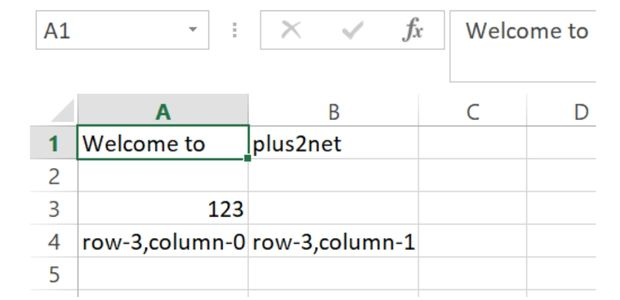``````import xlsxwriter
my_path='G:\\My drive\\testing\\xlsxwriter\\demo1.xlsx'#Path
wb = xlsxwriter.Workbook(my_path) # create workbook

ws.set_column('A:A',13) # increase width of the column
ws.set_column('B:B',13) # increase width of the column

ws.write('A1', 'Welcome to ')
ws.write('B1', 'plus2net')

# using row/column notation. Starting with row=0,column=0

ws.write(2,0, 123) # row 2 , column 0
ws.write(3,0, 'row-3,column-0')
ws.write(3,1, 'row-3,column-1')
wb.close()``````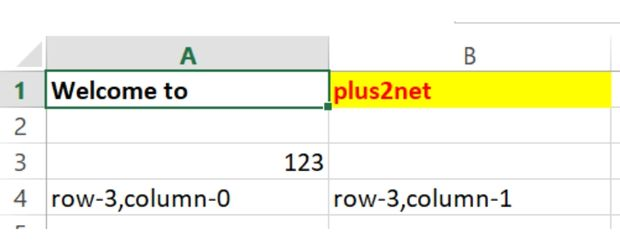``````bold = wb.add_format({'bold': True}) # Adding bold format
my_format = wb.add_format({'bold': True,
'font_color': 'red','bg_color':'#FFFF00'})
# Text with formatting.
ws.write('A1', 'Welcome to ',bold) # adding bold format
ws.write('B1', 'plus2net',my_format) # adding font and background color``````

## write() : Adding List to Excel file

``````import xlsxwriter
my_path='G:\\My drive\\testing\\xlsxwriter\\demo1.xlsx'
wb = xlsxwriter.Workbook(my_path) # create workbook

l1 = [1,2,3,4,5,6,7] # List to populate
r,c=0,0 # starting row and column

for data in l1:
ws.write(r,c,data)
r=r+1 # increase row value by 1
wb.close()``````
Using write_row() we can add the list in one row
``````import xlsxwriter
my_path='G:\\My drive\\testing\\xlsxwriter\\demo1.xlsx'
wb = xlsxwriter.Workbook(my_path) # create workbook

l1 = [1,2,3,4,5,6,7] # List to populate

ws.write_row('A1', l1) # Add list in one row
wb.close()``````

## write_formula()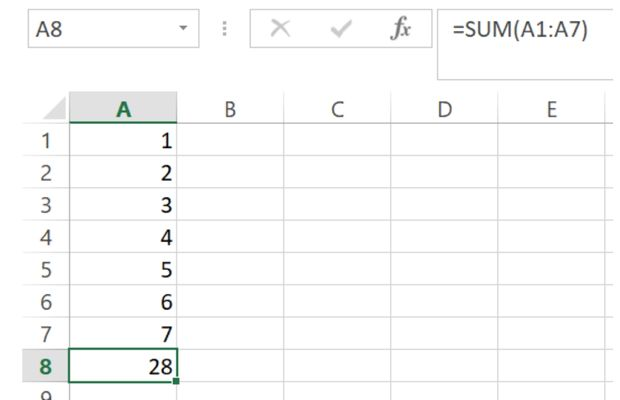Using formula, we will add all the cell values from A1 to A7 and display the same at A8
``````import xlsxwriter
my_path='G:\\My drive\\testing\\xlsxwriter\\demo1.xlsx'
wb = xlsxwriter.Workbook(my_path) # create workbook

l1 = [1,2,3,4,5,6,7] # List to populate
r,c=0,0 # starting row and column

for data in l1:
ws.write(r,c,data)
r=r+1 # increase row value by 1

ws.write_formula('A8', '=SUM(A1:A7)')
wb.close()``````

## Adding dictionary to Excel file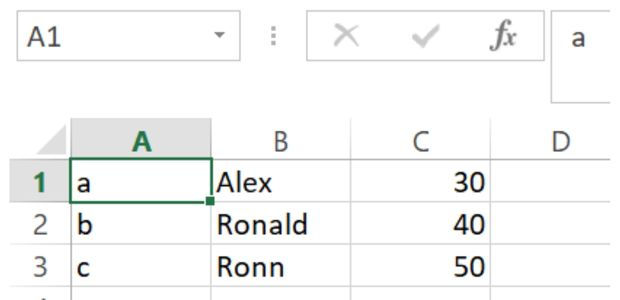``````import xlsxwriter
my_path='G:\\My drive\\testing\\xlsxwriter\\demo1.xlsx'
wb = xlsxwriter.Workbook(my_path) # create workbook

my_dict={'a':['Alex',30],'b':['Ronald',40],'c':['Ronn',50]}
r,c=0,0 # starting row and column

for k in my_dict:
ws.write(r,c,k) # adding Key at first column
ws.write_row(r,c+1,my_dict[k]) # adding List after key in same row
r=r+1 #increase row value by 1

wb.close()``````

## insert_image() : Adding image to Excel file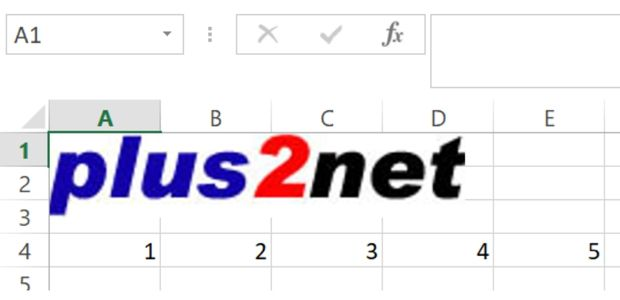``````import xlsxwriter
my_path='G:\\My drive\\testing\\xlsxwriter\\demo1.xlsx'
wb = xlsxwriter.Workbook(my_path) # create workbook

ws.insert_image('A1', 'D:\\top2.jpg') # Cell and Path of the image

l1 = [1,2,3,4,5] # List to populate

ws.write_row(3,0, l1) # Add list in one row
wb.close()``````

## insert_chart() : Adding chart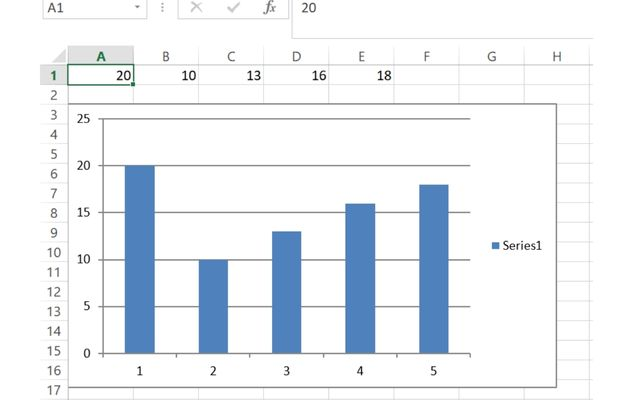``````import xlsxwriter
my_path='G:\\My drive\\testing\\xlsxwriter\\demo1.xlsx'
wb = xlsxwriter.Workbook(my_path) # create workbook
l1 = [20,10,13,16,18] # List to populate
ws.write_row(0,0, l1) # Add list in one row

chart = wb.add_chart({'type': 'column'}) # chart object
ws.insert_chart('A3', chart) # adding chart
wb.close()``````

Subscribe to our YouTube Channel here

## Subscribe

* indicates required
Subscribe to plus2netplus2net.com

Post your comments , suggestion , error , requirements etc here